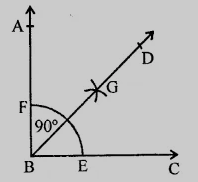# Using protractor, draw a right angle. Bisect it to get an angle of measure $45^o$.

To do:

We have to draw a right angle and bisect it to get an angle of measure $45^o$.

Solution:Steps of construction:

(i) Using a protractor, draw a right-angled triangle $ABC$.

$\angle ABC = 90^o$.

(ii) With centre $B$ and a suitable radius, draw an arc that meets $BC$ at $E$ and $BA$ at $F$.

(iii) With centre $E$ and $F,$ draw arcs intersecting each other at $G$.

(iv) Join $BG$ and produce it to $D$.

Therefore, $BD$ is the bisector of $\angle ABC$.

This implies,

$\angle DBC =\frac{1}{2}\times90^o$

$= 45^o$.

Updated on: 10-Oct-2022

24 Views